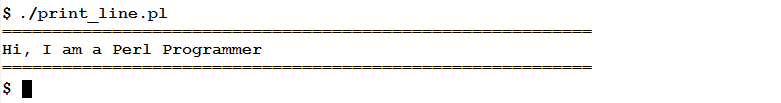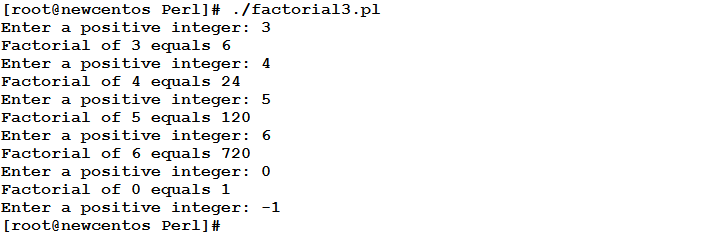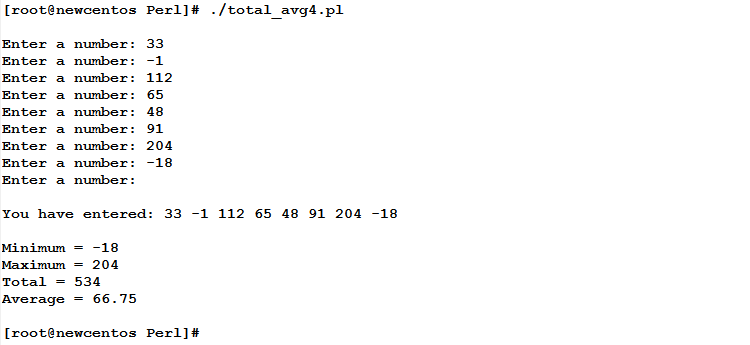# Learn about Subroutines in Perl Programming

1
7265What are Subroutines?
Subroutines (usually known as Functions in some other programming languages) are named blocks of code that achieve something, and are invoked (called) on need.

Why Subroutines?
Using subroutines is a neat alternative to writing the same code block everywhere a specific task is to be executed. Subroutines also increase code reusability. You wrote a script that defines three subroutines. When I read your script, I found that one or more of these subroutines could be useful to me in preparing a long script that was assigned to me to write. I could easily copy the functions (subroutines), and paste them on top of my program, and call them as the program logic dictates. The ability to reuse code and share it with others enriches the developing process.

Syntax

```sub FUNCTION_NAME
{
Statement(s) of the Subroutine Body
}
```

Where:
FUNCTION_NAME is the name of the subroutine. It could be any valid Perl identifier.

Example
The following script defines a subroutine that when called prints a line consisting of equal signs “=”

```#!/bin/perl
sub println
{
print ("===========================================================\n");
}
println();
print ("Hi, I am a Perl Programmer\n");
println();
```

When executed, this program should give the following output:To use the defined function, we need to call it as we did (twice) in the above script:

`println();`

Passing Arguments and Returning Values
A subroutine can accept input(s) from the calling program. These inputs are called arguments (parameters). The passed arguments are stored in a special array @_

Arguments could be:

• A group of scalars.
• One or more scalars and an array.
• An array.

If required, a subroutine can return value(s). The returned value(s) could be one scalar, or an array of scalars.

Example
The following script defines a function named factorial that receives one integer number as argument, and calculates the factorial for that number.

```#!/usr/bin/perl
sub factorial
{
\$result = 1;
foreach \$i (1..\$_)
{
\$result *= \$i;
}
return \$result;
}
while (true)
{
print ("Enter a positive integer: ");
\$num = <stdin>;
chomp \$num;
last if(\$num < 0);
\$r = factorial (\$num);
print("Factorial of \$num equals \$r\n");
}
```

Let’s see how this script will behave.In this script, I would like to explain a few points:

• The passed argument is stored in the @_ array as the first element \$_
• The calculated factorial value (the variable \$result) is returned back to the calling program using the return statement.
• As the calling program expects a return value, the program calls the factorial function and assigns that function call to a variable \$rExample
In this example, we are going to write a script that accepts a variable number of input numbers. When the user is done with entering input numbers (by entering an empty string to mark end of input), the script passes the numbers to a function that calculates their total, average, minimum, and maximum, and returns all the values to the main program.

Read the following script with me:

```#!/usr/bin/perl
#This script accepts a variable number of input numbers
#from the user, and passes them to a function that returns
#the total, average, minimum, and maximum.
#AUTHOR: Eduonix
#DATE: Dec 2015
sub calc
{
\$total = 0;
\$count = scalar (@_);
\$min = \$max = \$_;
foreach \$n (@_)
{
\$total += \$n;
\$min = \$n if (\$n < \$min);
\$max = \$n if (\$n > \$max);
}
return (\$total, \$total / \$count, \$min, \$max);
}
@numbers = ();
print("\nEnter a number: ");
until((\$i = <stdin>) == "")
{
chomp \$i;
push @numbers, \$i;
print("Enter a number: ");
}
print ("\nYou have entered: @numbers\n");
(\$t, \$a, \$minimum, \$maximum) = calc (@numbers);
print ("\nMinimum = \$minimum\n");
print ("Maximum = \$maximum\n");
print ("Total = \$t\n");
print ("Average = ",\$a,"\n\n");
```

When executed, the above script will behave as follows:In the above script, the main program passes an array (literally an array variable, not a list of scalars) as an argument to the subroutine. The subroutine has returned a list of scalars containing the calculated statistics.

Recursive Subroutines
A subroutine can recursively call itself. This should be done with extra care, so that the subroutine converges at the end. If not, the function will keep calling itself forever and the program crash.

Summary

• Subroutines (sometimes called methods or functions) are named blocks of code that achieve a task or make calculations.
• Subroutines could accept one or more arguments. (or take no arguments at all)
• Passed arguments are stored inside the subroutine definition in a special array variable @_
• A subroutine could return a scalar or an array.
• A subroutine can recursively call itself.

In the next article, we are going to learn how to read from and write to files. A very interesting topic to read. See you there!!

#### 1 COMMENT

1.XiddiXaid

Step by step guide , really valuable for me as a starter.